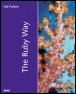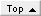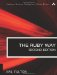# Working with HashesRuby Way By Hal Fulton Slots : 1.0 Table of Contents

Hashes are known in some circles as associative arrays, dictionaries, and by various other names. Perl and Java programmers in particular will be familiar with this data structure.

Think of an array as an entity that creates an association between index x and data item y. A hash creates a similar association, with at least two exceptions. First, for an array, x is always an integer; for a hash, it need not be. Second, an array is an ordered data structure; a hash typically has no ordering.

A hash key can be of any arbitrary type. As a side effect, this makes a hash a nonsequential data structure. In an array, we know that element 4 follows element 3; but in a hash, the key may be of a type that does not define a real predecessor or successor. For this reason (and others), there is no notion in Ruby of the pairs in a hash being in any particular order.

You may think of a hash as an array with a specialized index, or as a database "synonym table" with two fields, stored in memory. Regardless of how you perceive it, it is a powerful and useful programming construct.

#### Creating a New Hash

As with Array, the special class method [] is used to create a hash. The data items listed in the brackets are used to form the mapping of the hash.

Six ways of calling this method are shown here (note that hashes a1 through c2 will all be populated identically):

` `

` a1 = Hash.[]("flat",3,"curved",2) a2 = Hash.[]("flat"=>3,"curved"=>2) b1 = Hash["flat",3,"curved",2] b2 = Hash["flat"=>3,"curved"=>2] c1 = { "flat",3,"curved",2} c2 = { "flat"=>3,"curved"=>2} # For a1, b1, and c1: There must be # an even number of elements. `

Also, the class method new can take a parameter specifying a default value. Note that this default value is not actually part of the hash; it is simply a value returned in place of nil. Here's an example:

` `

` d = Hash.new         # Create an empty hash e = Hash.new(99)     # Create an empty hash f = Hash.new("a"=>3) # Create an empty hash e["angled"]          # 99 e.inspect            # { } f["b"]               # { "a"=>3}  (default value is                      #   actually a hash itself) f.inspect            # { } `

#### Specifying a Default Value for a Hash

The default value of a hash is an object that is referenced in place of nil in the case of a missing key. This is useful if you plan to use methods with the hash value that are not defined for nil. It can be assigned upon creation of the hash or at a later time using the default= method.

All missing keys point to the same default value object, so changing the default value has a side effect:

` `

` a = Hash.new("missing")  # default value object is "missing" a["hello"]               # "missing" a.default="nothing" a["hello"]               # "nothing" a["good"] << "bye"       # "nothingbye" a.default                # "nothingbye" `

The special instance method fetch raises an IndexError exception if the key does not exist in the Hash object. It takes a second parameter that serves as a default value. Also, fetch optionally accepts a block to produce a default value in case the key is not found. This is in contrast to default, because the block allows each missing key to have its own default. Here's an example:

` `

` a = { "flat",3,"curved",2,"angled",5} a.fetch("pointed")                   # IndexError a.fetch("curved","na")               # 2 a.fetch("x","na")                    # "na" a.fetch("flat") { |x| x.upcase}        # 3 a.fetch("pointed") { |x| x.upcase}     # "POINTED" `

#### Accessing and Adding Key/Value Pairs

Hash has class methods [] and []=, just as Array has; they are used much the same way, except that they accept only one parameter. The parameter can be any object, not just a string (although string objects are commonly used). Here's an example:

` `

` a = { } a["flat"] = 3        # { "flat"=>3} a.[]=("curved",2)    # { "flat"=>3,"curved"=>2} a.store("angled",5)  # { "flat"=>3,"curved"=>2,"angled"=>5} `

The method store is simply an alias for the []= method, both of which take two arguments, as shown in the example.

The method fetch is similar to the [] method, except that it raises an IndexError for missing keys. It also has an optional second argument (or alternatively a code block) for dealing with default values (see the section "Specifying a Default Value for a Hash"). Here's an example:

` `

` a["flat"]        # 3 a.[]("flat")     # 3 a.fetch("flat")  # 3 a["bent"]        # nil `

Suppose you are not sure whether the Hash object exists, and you would like to avoid clearing an existing hash. The obvious way is to check whether the hash is defined, as shown here:

` `

` unless defined? a   a={ } end a["flat"] = 3 `

Another way to do this is as follows:

` `

` a ||= { } a["flat"] = 3 `

The same problem can be applied to individual keys, where you only want to assign a value if the key does not exist:

` `

` a=Hash.new(99) a             # 99 a                # { } a ||= 5       # 99 a                # { } b=Hash.new b                # { } b             # nil b ||= 5       # 5 b                # { 2=>5} `

Note that nil may be used as either a key or an associated value:

` `

` b={ } b      # nil b=nil b         # { 3=>nil} b.nil? # true b.nil? # true b[nil]=5 b         # { 3=>nil,nil=>5} b[nil]    # 5 b[b]   # 5 `

#### Deleting Key/Value Pairs

Key/value pairs of a Hash object can be deleted using clear, delete, delete_if, reject, reject!, and shift.

Use clear to remove all key/value pairs. This is essentially the same as assigning a new empty hash, but it's marginally faster.

Use shift to remove an unspecified key/value pair. This method returns the pair as a two-element array (or nil if no keys are left):

` `

` a = { 1=>2, 3=>4} b = a.shift       # [1,2] # a is now { 3=>4} `

Use delete to remove a specific key/value pair. It accepts a key and returns the value associated with the key removed (if found). If the key is not found, the default value is returned. It also accepts a block to produce a unique default value rather than just a reused object reference. Here's an example:

` `

` a = { 1=>1, 2=>4, 3=>9, 4=>16} a.delete(3)                     # 9 # a is now { 1=>1, 2=>4, 4=>16} a.delete(5)                     # nil in this case a.delete(6) {  "not found" }      # "not found" `

Use delete_if, reject, or reject! in conjunction with the required block to delete all keys for which the block evaluates to true. The method reject uses a copy of the hash, and reject! returns nil if no changes were made.

#### Iterating over a Hash

The Hash class has the standard iterator each, as is to be expected. It also has each_key, each_pair, and each_value (each_pair is an alias for each). Here's an example:

` `

` { "a"=>3,"b"=>2} .each do |key, val|   print val, " from ", key, "; "    # 3 from a; 2 from b; end `

The other two provide only one or the other (the key or the value) to the block:

` `

` { "a"=>3,"b"=>2} .each_key do |key|   print "key = #{ key} ;"      # Prints: key = a; key = b; end { "a"=>3,"b"=>2} .each_value do |value|   print "val = #{ value} ;"    # Prints: val = 3; val = 2; end `

#### Inverting a Hash

Inverting a hash in Ruby is trivial with the invert method:

` `

` a = { "fred"=>"555-1122","jane"=>"555-7779"} b = a.invert b["555-7779"]     # "jane" `

Because hashes have unique keys, there is potential for data loss when doing thisduplicate associated values will be converted to a unique key using only one of the associated keys as its value. There is no predictable way to tell which one will be used.

#### Detecting Keys and Values in a Hash

Determining whether a key has been assigned can be done with has_key? or any one of its aliases: include?, key?, or member?. Here's an example:

` `

` a = { "a"=>1,"b"=>2} a.has_key? "c"        # false a.include? "a"        # true a.key? 2              # false a.member? "b"         # true `

You can also use empty? to see whether there are any keys at all left in the hash; length or its alias size can be used to determine how many there are, as shown here:

` `

` a.empty?        # false a.length        # 2 `

Alternatively, you can test for the existence of an associated value using has_value? or value?:

` `

` a.has_value? 2        # true a.value? 99           # false `

#### Extracting Hashes into Arrays

To convert the entire hash into an array, use the to_a method. In the resulting array, keys will be even-numbered elements (starting with 0) and values will be odd-numbered elements of the array:

` `

` h = { "a"=>1,"b"=>2} h.to_a        # ["a",1,"b",2] `

It is also possible to convert only the keys or only the values of the hash into an array:

` `

` h.keys         # ["a","b"] h.values       # [1,2] `

Finally, you can extract an array of values selectively based on a list of keys, using the indices method. This works for hashes much as the method of the same name works for arrays (the alias is indexes):

` `

` h = { 1=>"one",2=>"two",3=>"three",4=>"four","cinco"=>"five"} h.indices(3,"cinco",4)     # ["three","five","four"] h.indexes(1,3)             # ["one","three"] `

#### Selecting Key/Value Pairs by Criteria

The Hash class mixes in the Enumerable module, so you can use detect (find), select (find_all), grep, min, max, and reject as with arrays.

The detect method (whose alias is find) finds a single key/value pair. It takes a block (into which the pairs are passed one at a time) and returns the first pair for which the block evaluates to true. Here's an example:

` `

` names = { "fred"=>"jones","jane"=>"tucker",          "joe"=>"tucker","mary"=>"SMITH"} # Find a tucker names.detect { |k,v| v=="tucker" }     # ["joe","tucker"] # Find a capitalized surname names.find { |k,v| v==v.upcase }     # ["mary", "SMITH"] `

Of course, the objects in the hash can be of arbitrary complexity, as can the test in the block, but comparisons between differing types can cause problems.

The select method (whose alias is find_all) will return multiple matches, as opposed to a single match:

` `

` names.select { |k,v| v=="tucker" } # [["joe", "tucker"], ["jane", "tucker"]] names.find_all { |k,v| k.count("r")>0} # [["mary", "SMITH"], ["fred", "jones"]] `

#### Sorting a Hash

Hashes are by their nature not ordered according to the value of their keys or associated values. In performing a sort on a hash, Ruby converts the hash to an array and then sorts that array. The result is naturally an array:

` `

` names = { "Jack"=>"Ruby","Monty"=>"Python",          "Blaise"=>"Pascal", "Minnie"=>"Perl"} list = names.sort # list is now: # [["Blaise","Pascal"], ["Jack","Ruby"], #  ["Minnie","Perl"], ["Monty","Python"]] `

#### Merging Two Hashes

Merging hashes may be useful sometimes. Ruby's update method will put the entries of one hash into the target hash, overwriting any previous duplicates:

` `

` dict = { "base"=>"foundation", "pedestal"=>"base"} added = { "base"=>"non-acid", "salt"=>"NaCl"} dict.update(added) # { "base"=>"non-acid", "pedestal"=>"base", "salt"=>"NaCl"} `

#### Creating a Hash from an Array

The easiest way to create a hash from an array is to remember the bracket notation for creating hashes. This works if the array has an even number of elements. Here's an example:

` `

` array = [2, 3, 4, 5, 6, 7] hash = Hash[*array] # hash is now: { 2=>3, 4=>5, 6=>7} `

#### Finding Difference or Intersection of Hash Keys

Because the keys of a hash can be extracted as a separate array, the extracted arrays of different hashes can be manipulated using the Array class methods & and - to produce the intersection and difference of the keys. The matching values can be generated with the each method performed on a third hash representing the merge of the two hashes (to ensure all keys can be found in one place):

` `

` a = { "a"=>1,"b"=>2,"z"=>3} b = { "x"=>99,"y"=>88,"z"=>77} intersection = a.keys & b.keys difference = a.keys - b.keys c = a.dup.update(b) inter = { } intersection.each { |k| inter[k]=c[k] } # inter is { "z"=>77} diff={ } difference.each { |k| diff[k]=c[k] } # diff is { "a"=>1, "b"=>2} `

#### Using a Hash As a Sparse Matrix

Often we want to make use of an array or matrix that is nearly empty. We could store it in the conventional way, but this is often wasteful of memory. A hash provides a way to store only the values that actually exist.

Here is an example in which we are assuming that the nonexistent values should default to zero:

` `

` values = Hash.new(0) values = 5 values = 7 values = 9 x = values      # 9 y = values      # 0 `

Obviously in this example, an array would have created over 9,000 unused elements. This may not be acceptable.

What if we want to implement a sparse matrix of two or more dimensions? All we need do is use arrays as the hash keys, like so:

` `

` cube = Hash.new(0) cube[[2000,2000,2000]] = 2 z = cube[[36,24,36]]       # 0 `

In this case, we see that literally billions of array elements would need to be created if this three-dimensional array were to be complete.

#### Implementing a Hash with Duplicate Keys

Purists would likely say that if a hash has duplicate keys, it isn't really a hash. We don't want to argue. Call it what you will, there might be occasions when you want a data structure that offers the flexibility and convenience of a hash but allows duplicate key values.

We offer a partial solution here (see Listing 3.9). It is partial for two reasons. First, we have not bothered to implement all the functionality that could be desired, but only a good representative subset. Second, the inner workings of Ruby are such that a hash literal is always an instance of the Hash class, and even though we were to inherit from Hash, a literal would not be allowed to contain duplicates. (We're thinking about this one further.)

But as long as you stay away from the hash-literal notation, this problem is doable. Here we implement a class that has a "store" (@store) that is a simple hash; each value in the hash is an array. We control access to the hash in such a way that when we find ourselves adding a key that already exists, we add the value to the existing array of items associated with that key.

What should size return? Obviously, the "real" number of key/value pairs including duplicates. Likewise, the keys method returns a value potentially containing duplicates. The iterators behave as expected; as with a normal hash, there is no predicting the order in which the pairs will be visited.

Besides the usual delete, we have implemented a delete_pair method. The former will delete all values associated with a key; the latter will delete only the specified key/value pair. (Note that it would have been difficult to make a single method such as delete(k,v=nil) because nil is a valid value for any hash.)

For brevity, we have not implemented the entire class; frankly, some of the methods, such as invert, would require some design decisions as to what their behavior should be. If you're interested, you can flesh out the rest as needed.

##### Listing 3.9 Hash with Duplicate Keys
` class HashDup   def initialize(*all)     raise IndexError if all.size % 2 != 0     @store = { }     if all  # not nil       keyval = all.dup       while !keyval.empty?         key = keyval.shift         if @store.has_key?(key)           @store[key] += [keyval.shift]         else           @store[key] = [keyval.shift]         end       end     end   end   def store(k,v)     if @store.has_key?(k)       @store[k] += [v]     else       @store[k] = [v]     end   end   def [](key)     @store[key]   end   def []=(key,value)     self.store(key,value)   end   def to_s     @store.to_s   end   def to_a     @store.to_a   end   def inspect     @store.inspect   end   def keys     result=[]     @store.each do |k,v|       result += ([k]*v.size)     end     result   end   def values     @store.values.flatten   end   def each     @store.each { |k,v| v.each { |y| yield k,y} }   end   alias each_pair each   def each_key     self.keys.each { |k| yield k}   end   def each_value     self.values.each { |v| yield v}   end   def has_key? k     self.keys.include? k   end   def has_value? v     self.values.include? v   end   def length     self.values.size   end   alias size length   def delete k     val = @store[k]     @store.delete k     val   end   def delete k,v     @store[k] -= [v] if @store[k]     v   end   # Other methods omitted here... end # This won't work... dup key will ignore # first occurrence. h = { 1=>1, 2=>4, 3=>9, 4=>16, 2=>0} # This will work... h = HashDup.new(1,1, 2,4, 3,9, 4,16, 2,0) k = h.keys         # [4, 1, 2, 2, 3] v = h.values       # [16, 1, 4, 0, 9] n = h.size         # 5 h.each { |k,v| puts "#{ k}  => #{ v} "} # Prints: # 4 => 16 # 1 => 1 # 2 => 4 # 2 => 0 # 3 => 9 `The Ruby Way, Second Edition: Solutions and Techniques in Ruby Programming (2nd Edition)
ISBN: 0672328844
EAN: 2147483647
Year: 2000
Pages: 119
Authors: Hal Fulton

Similar book on Amazon